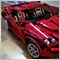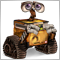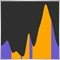# How can I code that a moving average is pointing in any direction?1264

Hello!

I'm trying to make a system based on 3 SMA. I want to take a trade if all 3 SMA are pointing in the same direction (up or down), but I'm struggling on how to represent that in a code.

I thought that it might be a good idea using a % change and set a bias, so if all the values are above the bias, it will be a valid.

Another approach (wich I have not tested yet) is to calculate the angle with tanh function. I imagined the points of the SMA as the hypotenuse of small triangles. With that said, the lenght of one side would be the difference between sma on bar 1 and sma on bar 2

and the other I guess that it would be just 1 (because its from 1 bar to the other bar). Is this approach correct?

This is the part of the code that I'm actually using. Any idea or suggestion of how I can do it better will be appreciated!

PD: ema30Threshold is an input that represents the % of change, ie: 0.0001.

```   CopyBuffer(ema30Handle,0,0,40,ema30Buffer);
CopyBuffer(ema50Handle,0,0,40,ema50Buffer);
CopyBuffer(ema100Handle,0,0,40,ema100Buffer);

int varAux4 = 0; //Variable auxiliar para ver que la 30 SMA apuntan en la misma direccion
int varAux5 = 0; //Variable auxiliar para ver que la 50 SMA apuntan en la misma direccion
int varAux6 = 0; //Variable auxiliar para ver que la 100 SMA apuntan en la misma direccion

for(int i=0;i<ema30Barsup;i++)
{
if(((ema30Buffer[i]-ema30Buffer[i+1])/ema30Buffer[i+1])*100>ema30Threshold && ema30Buffer[i]>ema30Buffer[i+1] && varAux4==0) //checkear para uptrend
{
varAux4==1;
}
if((((ema30Buffer[i]-ema30Buffer[i+1])/ema30Buffer[i+1])*100<=ema30Threshold || ema30Buffer[i]<ema30Buffer[i+1]) && varAux4==1)
{
varAux4==0;
break;
}
if(((ema30Buffer[i]-ema30Buffer[i+1])/ema30Buffer[i+1])*100<ema30Threshold && ema30Buffer[i]<ema30Buffer[i+1] && varAux4==0) //checkear para downtrend
{
varAux4==-1;
}
if((((ema30Buffer[i]-ema30Buffer[i+1])/ema30Buffer[i+1])*100>=ema30Threshold || ema30Buffer[i]>ema30Buffer[i+1]) && varAux4==-1)
{
varAux4==0;
break;
}
}

for(int i=0;i<ema50Barsup;i++)
{
if(((ema50Buffer[i]-ema50Buffer[i+1])/ema50Buffer[i+1])*100>ema50Threshold && ema50Buffer[i]>ema50Buffer[i+1] && varAux5==0) //checkear para uptrend
{
varAux5==1;
}
if((((ema50Buffer[i]-ema50Buffer[i+1])/ema50Buffer[i+1])*100<=ema50Threshold || ema50Buffer[i]<ema50Buffer[i+1]) && varAux5==1)
{
varAux5==0;
break;
}
if(((ema50Buffer[i]-ema50Buffer[i+1])/ema50Buffer[i+1])*100<ema50Threshold && ema50Buffer[i]<ema50Buffer[i+1] && varAux5==0) //checkear para downtrend
{
varAux5==-1;
}
if((((ema50Buffer[i]-ema50Buffer[i+1])/ema50Buffer[i+1])*100>=ema50Threshold || ema50Buffer[i]>ema50Buffer[i+1]) && varAux5==-1)
{
varAux5==0;
break;
}
}

for(int i=0;i<ema100Barsup;i++)
{
if(((ema100Buffer[i]-ema100Buffer[i+1])/ema100Buffer[i+1])*100>ema100Threshold && ema100Buffer[i]>ema100Buffer[i+1] && varAux6==0) //checkear para uptrend
{
varAux6==1;
}
if((((ema100Buffer[i]-ema100Buffer[i+1])/ema100Buffer[i+1])*100<=ema100Threshold || ema100Buffer[i]<ema100Buffer[i+1]) && varAux6==1)
{
varAux6==0;
break;
}
if(((ema100Buffer[i]-ema100Buffer[i+1])/ema100Buffer[i+1])*100<ema100Threshold && ema100Buffer[i]<ema100Buffer[i+1] && varAux6==0) //checkear para downtrend
{
varAux6==-1;
}
if((((ema100Buffer[i]-ema100Buffer[i+1])/ema100Buffer[i+1])*100>=ema100Threshold || ema100Buffer[i]>ema100Buffer[i+1]) && varAux6==-1)
{
varAux6==0;
break;
}
}```1775

I think if your intent is to go through bar by bar to determine whether the magnitude of MA change implies an Up or a Down direction, your current approach (as coded) is good enough, no need to calculate angle/tanh. Angle/tanh may only be useful if you want to deal with a number of bars collectively (i.e. computing the angle formed by MA changes over N bars as a whole, for example).

However I do see some bugs in your code, hope you've figured them out already.1264

Seng Joo Thio:

I think if your intent is to go through bar by bar to determine whether the magnitude of MA change implies an Up or a Down direction, your current approach (as coded) is good enough, no need to calculate angle/tanh. Angle/tanh may only be useful if you want to deal with a number of bars collectively (i.e. computing the angle formed by MA changes over N bars as a whole, for example).

However I do see some bugs in your code, hope you've figured them out already.

Hi Seng,

Thank you for your reply! Yeah, the ange part makes sense when you compare it with the number of bars. You said that my current approach as coded is good enough. How would you code it?

And yes, I noticed some bugs, lol. varAux==1 isn't going to take me anywhere haha.

If you have anything else to add I will greatly appreciate it!

Regards,

PS1775

Pedro Severin:

Hi Seng,

Thank you for your reply! Yeah, the ange part makes sense when you compare it with the number of bars. You said that my current approach as coded is good enough. How would you code it?

And yes, I noticed some bugs, lol. varAux==1 isn't going to take me anywhere haha.

If you have anything else to add I will greatly appreciate it!

Regards,

PS

Right... when I said "good enough" I was referring just to the direction determination conditions in general, which does give you what you want. E.g.:

`((ema30Buffer[i]-ema30Buffer[i+1])/ema30Buffer[i+1])*100>ema30Threshold && ema30Buffer[i]>ema30Buffer[i+1]`

And when I mentioned "some bugs", there were more bugs than just varAux==1 alone.

Ok, let's look at them one by one:

(1) For loops - not necessary since u'r just trying to determine the current direction. So using 1 and 2 will do, rather than i and i+1. Also, the statements within each of the for loop will return their corresponding varAux as 0 (after fixing the == bug) since as u move to earlier candles, sooner or later you'll get to a point where the direction checks result in 0 and break from the loop.

(2) Notice that when the direction is down, "ema30Buffer[i]-ema30Buffer[i+1])/ema30Buffer[i+1])*100" will be negative, and comparing with the same threshold as when the direction is up won't do you much good. Should consider using MathAbs to remove the negative sign and always check if it's greater than threshold regardless of direction.12409

Pedro Severin:

Hello!

I'm trying to make a system based on 3 SMA. I want to take a trade if all 3 SMA are pointing in the same direction (up or down), but I'm struggling on how to represent that in a code.

I thought that it might be a good idea using a % change and set a bias, so if all the values are above the bias, it will be a valid.

Another approach (wich I have not tested yet) is to calculate the angle with tanh function. I imagined the points of the SMA as the hypotenuse of small triangles. With that said, the lenght of one side would be the difference between sma on bar 1 and sma on bar 2

and the other I guess that it would be just 1 (because its from 1 bar to the other bar). Is this approach correct?

This is the part of the code that I'm actually using. Any idea or suggestion of how I can do it better will be appreciated!

PD: ema30Threshold is an input that represents the % of change, ie: 0.0001.1264

Seng Joo Thio:

Right... when I said "good enough" I was referring just to the direction determination conditions in general, which does give you what you want. E.g.:

And when I mentioned "some bugs", there were more bugs than just varAux==1 alone.

Ok, let's look at them one by one:

(1) For loops - not necessary since u'r just trying to determine the current direction. So using 1 and 2 will do, rather than i and i+1. Also, the statements within each of the for loop will return their corresponding varAux as 0 (after fixing the == bug) since as u move to earlier candles, sooner or later you'll get to a point where the direction checks result in 0 and break from the loop.

(2) Notice that when the direction is down, "ema30Buffer[i]-ema30Buffer[i+1])/ema30Buffer[i+1])*100" will be negative, and comparing with the same threshold as when the direction is up won't do you much good. Should consider using MathAbs to remove the negative sign and always check if it's greater than threshold regardless of direction.

1. For the loops I use the last 3 values of the SMA. I wanted to know if I should use more, that's why the loop, but it still doesn't fully convince me. I mean, I don't know if it is well written and I'm getting what I really want.

2. Yes, thank you for pointing that. I corrected it and added to the code :)1264

Marco Montemari:

Hi Marco!

And how should I use the channels in this logic?21863

1. Seng Joo Thio: no need to calculate angle/tanh. Angle/tanh

2. Pedro Severin:I want to take a trade if all 3 SMA are pointing in the same direction (up or down), but I'm struggling on how to represent that in a code.
It's pointing up if the current value is higher than a previous one.12409

Pedro Severin:

Hi Marco!

And how should I use the channels in this logic?

Open a chart and add 2 regressional channels having different length....after observing it will be clear to you ;)1264

William Roeder:
1. It's pointing up if the current value is higher than a previous one.

1. I see your point, and you might be right, but I think what Seng suggested was if I need to calculate an angle, the most correctly approach would be that one. And by the way (correct me if I'm wrong), if I am on a daily chart and I want to calculate the angle of the 2 past bars (not the actual one), I could do it taking the base of the triangle as the distance between bars (which should be 1, right?) and then the height would be the high of the yesterday's bar minus the low of the day before yesterday's bar. That height shouldn't be fixed no matter what timefrime I look? Shouldn't the only variable remaining be the lenght of the base of the triangle, depending on the timeframe I look?

2. By the way, when you said "30 miles in 45 minutes" thats speed and that gave me an idea. Is there a way to measure "the speed of pips"? Like its changing at X speeds per minute/hour/day or any kind of time? Isn't ROC measuring that?

3. Yeah, its pointing up if the current value is higher than previous one, but what I want is to determine "how up" the SMA needs to be in order to consider it a healthy trend.

4. At last, but not least, I'm trying to code a pullback, regarding if it is a pullback to a SMA or not. How can I do this? It seems harder than it looks (or I'm missing something). I tried different ways, but it doesn't seems to work well. How would you do it?2469

Pedro Severin:

Hello!

I'm trying to make a system based on 3 SMA. I want to take a trade if all 3 SMA are pointing in the same direction (up or down), but I'm struggling on how to represent that in a code.

I thought that it might be a good idea using a % change and set a bias, so if all the values are above the bias, it will be a valid.

Another approach (wich I have not tested yet) is to calculate the angle with tanh function. I imagined the points of the SMA as the hypotenuse of small triangles. With that said, the lenght of one side would be the difference between sma on bar 1 and sma on bar 2

and the other I guess that it would be just 1 (because its from 1 bar to the other bar). Is this approach correct?

This is the part of the code that I'm actually using. Any idea or suggestion of how I can do it better will be appreciated!

PD: ema30Threshold is an input that represents the % of change, ie: 0.0001.

Hello Pedro
You can simply use the slope of your EMAs and check if the price is completely above or below the slowest EMA.